Mandelbrot Orbits
MANDELBROT ORBITS

Balmoral Software

### Introduction

The Mandelbrot set M is defined as those points c in the complex plane for which the complex sequence
 zn+1 = z2n + c, z0 = c (1)
converges; that is, |zn| is finite as n → ∞. It is well known that |zn| will eventually diverge if it ever exceeds 2 . Since z0 = c, it follows that all points in M are contained within a circle of radius 2 centered at the origin. Points in M are shown in black in the image below:The largest areas of M are a cardioid having its left edge at -3/4 and its cusp at 1/4, and a circle of radius 1/4 centered at (-1,0). Other regions of M include smaller near-circular "buds"  tangent to the main cardioid or to each other, as well as fractal filaments extending into other areas of the complex plane.

### Orbits

The values of {zn} in the sequence (1) form the orbit associated with the starting point c. The sequence converges to a single fixed point when c is on or inside the boundary of the main cardioid . If c is in one of the buds, zn converges to a repeating cycle of two or more points , with the length of the cycle, or period, determined by which bud c is in.

The orbit of a starting point that is within the Mandelbrot set does not itself need to consist of points inside the set; for example, the orbit of

 c = -0.1225611668766536 - 0.7448617666197442i, (2)
immediately cycles amongst the three values
 z0 = -0.1225611668766536 - 0.7448617666197442i z1 = -0.6623589786223729 - 0.5622795120623012i z2 = 0, (3)
so c is in the Mandelbrot set but z1 is not since starting the orbit at z1 results in divergence in (1) after about six iterations. This period-3 cycle is shown as a brown triangle in the diagram below.

The value of c above was found by equating it to the result of three iterations of (1):

z0 = c

z1 = z20 + c = c2 + c

z2 = z21 + c = (c2 + c)2 + c = c4 + 2c3 + c2 + c

z3 = z22 + c = z0 = c

z2 = 0

Ignoring the trivial solution c = 0, we have
c3 + 2c2 + c + 1 = 0,
which has complicated closed-form solutions approximated by
c = -1.7548776662466928 + 0i,
(2), and its conjugate. The repeating cycle corresponding to the first point remains on the real axis:
z0 = -1.7548776662466928
z1 = 1.3247179572447461
z2 = 0
and the cycle corresponding to the second point is given in (3) above.

The diagram below is an approximation to the boundary of M , in which orbits of other sample points in the Mandelbrot set are shown in color with starting squares representing c: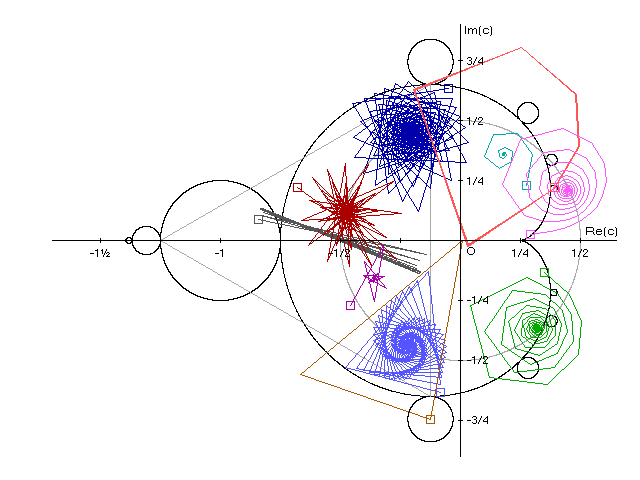In clockwise order starting near the top of the cardioid, these orbits are:

 c Color Orbit Dark blue -0.05 + 0.6333i Converges in a weblike pattern to the stationary point -0.2074 + 0.4476i Cyan 0.2767 + 0.23i Quickly converges in a small spiral to the stationary point 0.18 + 0.3593i Orange 0.39 + 0.22i Starting at the center of one of the main cardioid's east bulbs, cycles between 6 values inside and outside M Pink 0.29 + 0.0233i Smooth spiral converging on the stationary point 0.4439 + 0.2077i, which is outside the main cardioid of M Green 0.35 - 0.1333i From a point near the boundary of the main cardioid, spirals in to the stationary point 0.3175 + 0.3651i Brown -0.1267 - 0.7467i Starting at the center of the south bulb, oscillates between 3 values, one in the bulb, the other near the origin, and the third outside M Medium blue -0.0833 - 0.6333i Again starting near the main cardioid boundary, spirals in to the stationary point -0.2242 - 0.4372i Purple -0.46 - 0.27i Converges in a small starlike pattern to the stationary point -0.3572 - 0.1575i Dark gray -0.8433 + 0.0867i Starting in the largest bulb at left, oscillates between two values in M Red -0.68 + 0.22i From the left side of the main cardioid, converges in a large starburst pattern to the stationary point -0.4710 + 0.1133i

### Stationary Points

The orbit of a point c in the main cardioid of M converges to a single fixed (stationary) point z  when
 z = z2 + c (11)
and
 |z| ≤ 1/2 (4)
A value z satisfying (4) lies within a circle S of radius 1/2 centered at the origin, shown in light gray on the diagram above. The first expression above is a quadratic with solution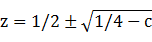(5)
Let the principal square root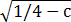be denoted by a + bi for real a and b. By definition, a ≥ 0. Ignoring the trivial case a = b = 0, for which c = 1/4 at the cusp of the main cardioid and z = 1/2, we have a > 0 and/or b ≠ 0. Therefore,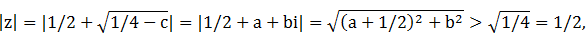so (4) is not satisfied. As a result, we can ignore the positive root in (5) and the stationary point z associated with c is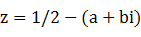Closed-form solution for the stationary point

If we let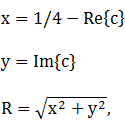we can solve for a and b algebraically: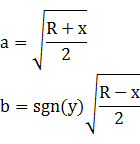For example, let c = -0.68 + 0.22i (red orbit in figure above):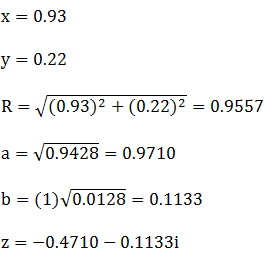Boundary mapping

It's easy to see that if c is on the boundary of the main cardioid, its stationary point z occurs on S.

Proof. A point c on the boundary of the main cardioid of M is defined by

Re{c} = [1 - cos(t)]cos(t)/2 + 1/4

Im{c} = [1 - cos(t)]sin(t)/2,

which reduces to
c = eit/2 - e2it/4
Substituting for the negative root in (5), we have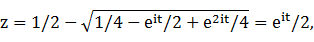so |z| = 1/2, QED.

Cardioid location test

A point z = a + bi is on or inside the main cardioid of M if and only if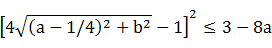(6)
Proof. It's simpler to compare z with the cardioid boundary after translation. With respect to the origin of M, translate the main cardioid to the left by 1/4 so that it can be represented by the polar function
r(t) = [1 - cos(t)]/2, 0 ≤ t < 2π
Similarly, translate the given point z to the left by 1/4, denoting the transformed value by w:
w = (a - 1/4) + bi
The distance of w from the origin isMeasured counterclockwise from the positive real axis, the radial angle associated with w is
θ = Atan2(b,a - 1/4)
The distance of the translated cardioid from the origin along a line to w is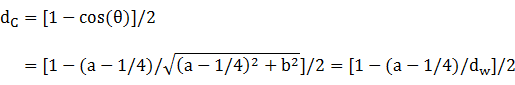It follows that the original point z is on or inside the Mandelbrot main cardioid if and only if
dw ≤ dC

2dw ≤ 1 - (a - 1/4)/dw,

which simplifies to
(4dw - 1)2 ≤ 3 - 8a, QED.
This formula is equivalent to those in Wikipedia.

Mandelbrot set regions

There can be orbits that converge on points outside the main cardioid of M, as shown in light blue in the diagram below. Likewise, stationary points cannot occur within the green areas of the main cardioid since they are outside S: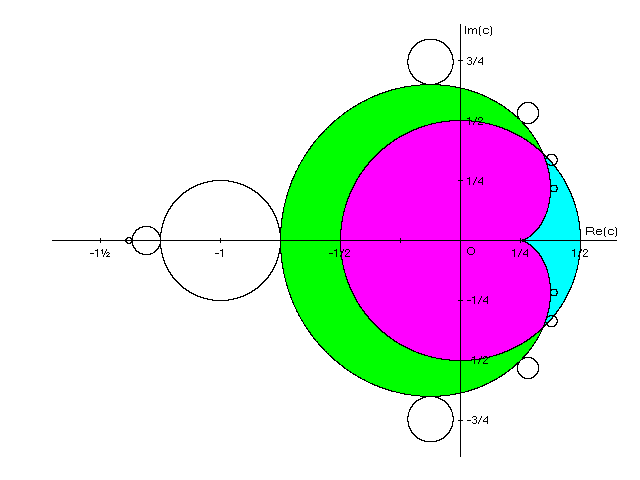For example, applying (6) to the starting point c = 0.29 + 0.0233i near the cusp of the main cardioid, we have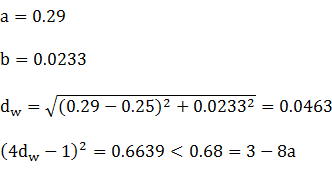Therefore, c is just inside the main cardioid, and also inside S since |c| = 0.2909 < 1/2. Then applying (6) to the stationary point z = 0.4439136 + 0.2077154i of c, we have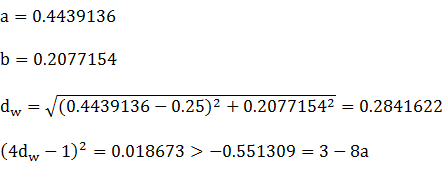so z is outside the main cardioid. The orbit from c to z is shown in pink in the preceding diagram.

### Other observations

The main cardioid of M has its maximum abscissa at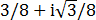and its maximum ordinate at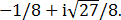To determine the intersection of the main cardioid and S, in the implicit formula for the cardioid, substitute 1/2 for a and x - 1/4 for x, then solve simultaneously with the equation for S:

[(x - 1/4)2 + y2 + (x - 1/4)/2]2 = [(x - 1/4)2 + y2]/4
and
x2 + y2 = 1/4
The solutions are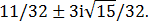In terms of area, most of the Mandelbrot set consists of its main cardioid together with its buds. In fact, the main cardioid plus its largest bud centered at -1 on the real axis together account for roughly 80-90% of the area of M (the exact area of M is unknown but bounded) .

The leftmost point P of the largest bud and the top and bottom points Q and R of the main cardioid form an equilateral triangle, as shown in light gray in the diagram above: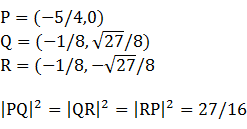Asymmetric or "deformed" Mandelbrot cardioids can occur in minibrots deep within the boundary of M . For example, consider a point c in the Seahorse Valley separating the main cardioid and largest bud of M:
c = -0.74583256875 + 0.1100764125i
Apply a magnification of 4000 and rotate the image counterclockwise by 105° to obtain the following image:### References

 Munafo, R., "Escape Radius", Usenet posting by Mike Hurley, 1993.

 Vepstas, L., "Mandelbrot Bud Maths", 2000.

 Cross, D., "Algebraic solution of Mandelbrot orbital boundaries", 2005.

 Devaney, R., "The Fractal Geometry of the Mandelbrot Set".

 Majewski, A., Wikibooks "Mandelbrot set components", 2008.

 Bittner, D., et. al., "New Approximations for the Area of the Mandelbrot Set", Involve: A Journal of Mathematics, 10(4) 555-572, 2017.

 Davis, A., et. al., "Warped Midgets in the Mandelbrot Set", Computers & Graphics, Vol. 18, Issue 2, 1994, reprinted in Pickover, C. (ed.), Chaos and Fractals: A Computer Graphical Journey, 1998: Elsevier Science B.V.